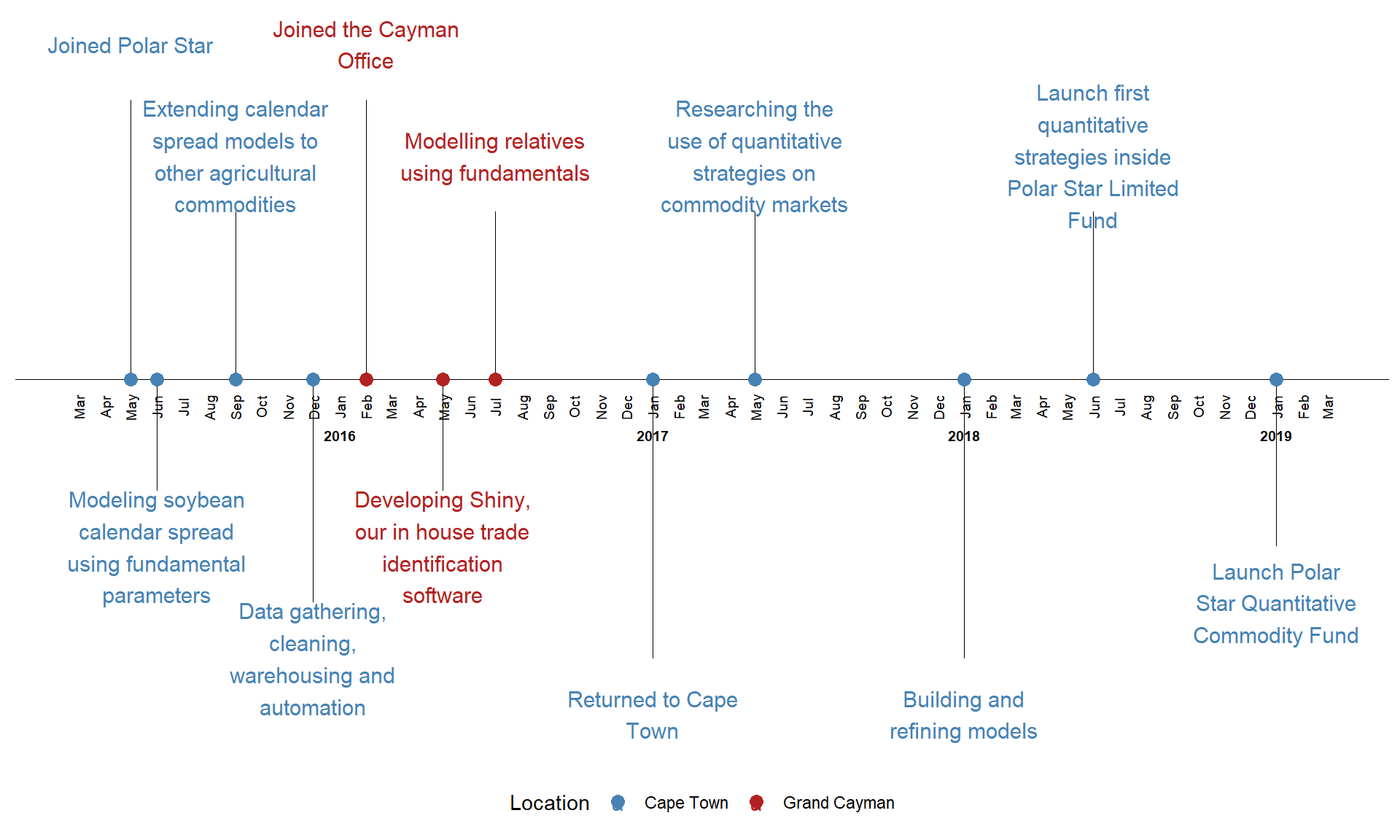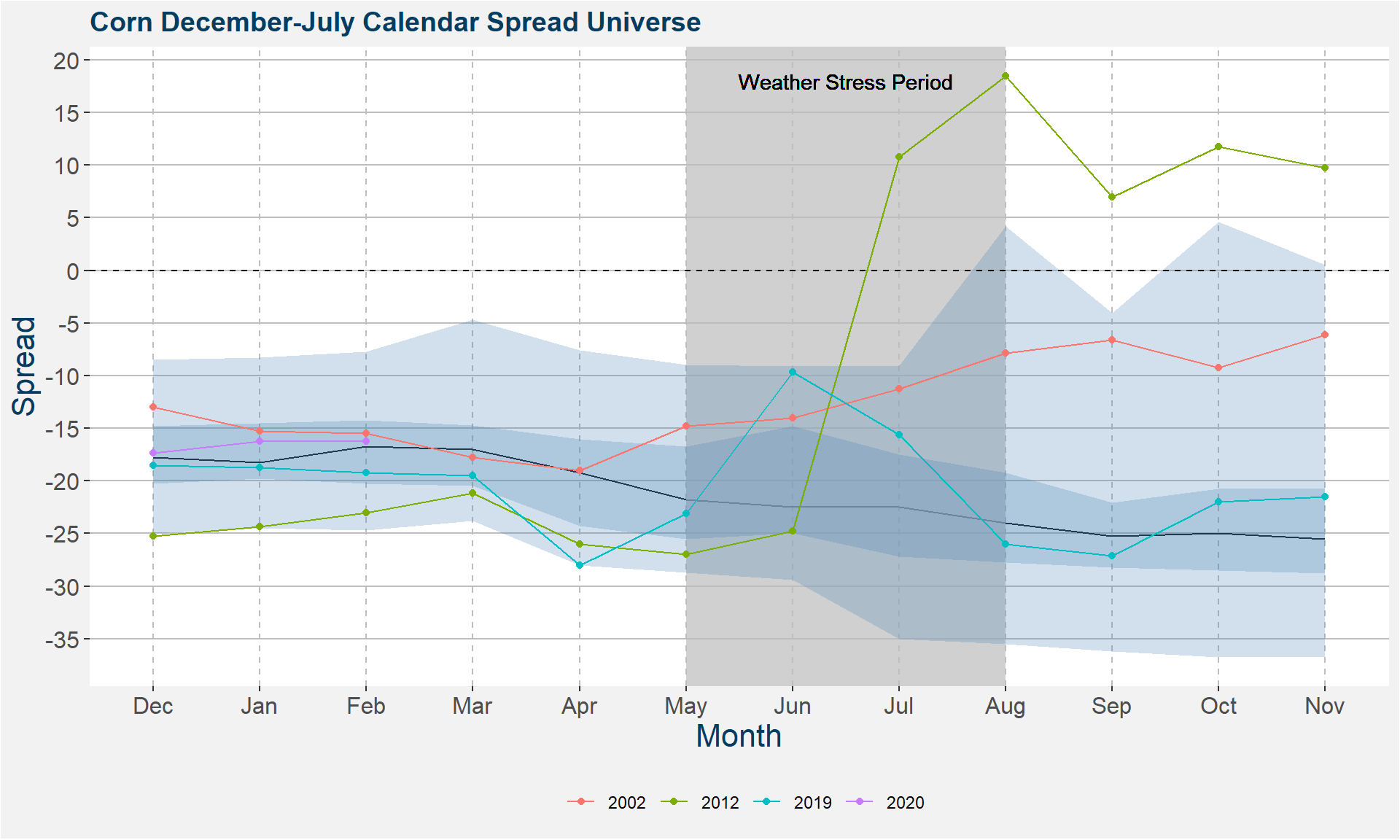# Polar Star Quantitative Commodity Fund

### 22 August, 2019

How has the increase of Automated Trading Systems (ATS) influenced the futures market?

• CME transaction data identitifies ATS with the 1028 tag
• Data available from November 2012 to present
• Consider data form 2012 to 2016
• Use gradient plots to highlight the changes
• Graphs are interactive

## Where are the opportunities?

• Limited, Local, Spectrum
• Less liquid parts of the curve
• Changes to macro
• Concentrated risk on asymmetric risk/reward trades

• Quantitative
• Harvest systematic alpha from large universe of commodities
• Rule based decision making process
• Strategies inspired by discretional methodology

# Grinold’s Fundamental Law of Active Portfolio Management

## Law of active portfolio management

$\text{Performance} = \text{Skill} \times \sqrt{\text{Breadth}}$

## Suppose we are in a coin flipping casino

• Flip coins in stead of futures
• The coin is biased - $$P(\text{heads}) = 0.51$$

## How does the betting work?

• We have 1000 coins
• The minimum wager is 1 coin
• If you win you gain 1 coin
• If you loose you loose 1 coin
• There are 1000 tables with coin wagers
• Games runs in parallel

## What is the optimal way to allocate coins?

Two extremes

• Bet 1000 coins on one coin flip
• Bet 1 coin on 1000 coin flips

## Expected Return

• Single bet: $$0.51 \times 1000 + 0.49 \times (-1000) = 20$$

• Multi bet: $$1000 \times [0.51 + 0.49 \times (-1)] = 20$$

The same expected return

## Risk - Probability to lose it all:

• Single bet: 49%

• Multi bet: $$0.49 \times 0.49 \times \dots \times 0.49 = 0.49^{1000} \approx 0$$

## Risk - Standard Deviation:

• One coin per table

$\text{risk} := \text{std}\left\{1,-1,-1,1, \dots, 1 \right\} = 1$

• One 1000 coin bet, 999 zero coin bets

\begin{align} \text{risk} &:= \text{std}\left\{1000,0,0,0, \dots, 0 \right\} = 31.62 \\ \text{risk} &:= \text{std}\left\{-1000,0,0,0, \dots, 0 \right\} = 31.62 \end{align}

## Coin Flip Casino - Reward/Risk

• Just like Sharpe Ratio

• Single bet: $$\text{SR}_{\text{single}} = \frac{20}{31.62} =0.63$$

• Multi bet: $$\text{SR}_{\text{multiple}} = \frac{20}{1} =20$$

## Coin flipping casino - Observation

• $$20 = 0.63 \times \sqrt{1000}$$

• $$\text{SR}_{\text{multiple}} = \text{SR}_{\text{single}} \times \sqrt{\text{Bets}}$$

• $$\text{Performance} = \text{Skill} \times \sqrt{\text{Breadth}}$$

## How does this apply to commodity futures?

• We use insights gained from years of fundamental trading to inspire bespoke quantitative strategies that are applied to a large collection of commodity markets

• We increase breadth or diversification by
• how,
• what and# Technical considerations when trading futures systematically

• Continuous Futures Price Series
• We require long time series data
• Futures expire too soon to gather sufficient data
• How do you handle rolls?

• Non-stationarity of Price Data
• Time series data can only reliably be forecasted if stationary
• Machine Learning algorithms are designed for stationary features
• How do we create stationary data?